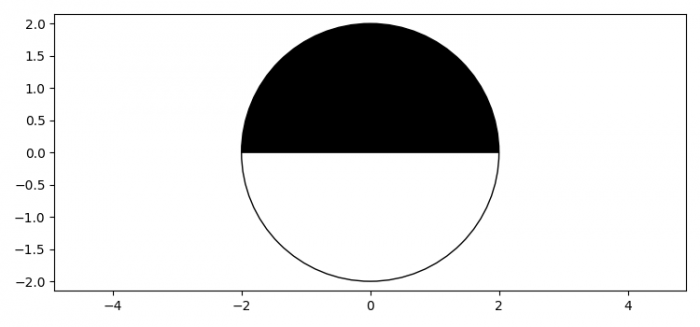# How to plot a half-black and half-white circle using Matplotlib?

To plot a half-black and half-white circle using Matplotlib, we can take the following steps −

• Set the figure size and adjust the padding between and around the subplots.
• Create a figure and a set of subplots.
• Initialize theta1 and theta2 to draw edges from theta1 to theta2 and vice-versa.
• Add the wedge instance on the current axes.
• Set equal scaling by changing axis limits.
• Set x and y scale.
• To display the figure, use show() method.

## Example

import matplotlib.pyplot as plt
from matplotlib.patches import Wedge

plt.rcParams["figure.figsize"] = [7.50, 3.50]
plt.rcParams["figure.autolayout"] = True

fig, ax = plt.subplots()
theta1, theta2 = 0, 0 + 180
center = (0, 0)
w1 = Wedge(center, radius, theta1, theta2, fc='black', edgecolor='black')
w2 = Wedge(center, radius, theta2, theta1, fc='white', edgecolor='black')

for wedge in [w1, w2]:
plt.show()